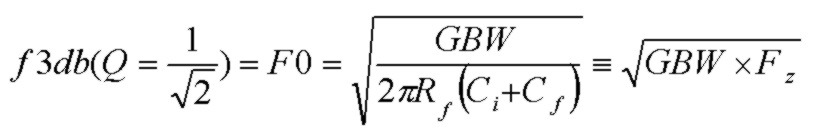# Transimpedance f3db and Cf Design Curves for Maximally Flat Frequency Response

Aug 10, 2012

The design curves below show transimpedance f3db bandwidth as a function of feedback resistance Rf for a range of op-amp GBW products with Cf chosen to satisfy the maximally flat frequency response condition of Q = 1/Sqrt(2) = 0.7071. Expressions for f3db and Cf(Q=0.7071) are provided below. Each set of curves is for a fixed total input capacitance Ci. The basic transimpedance circuit configuration is shown below with Ri taken as infinite (see expression at bottom of this page for result including Ri). The op-amp is assumed ideal except for the finite GBW product described by a single-pole open-loop gain function. Ci is the total input capacitance at the inverting input (sum of op-amp differential and common mode capacitances plus any stray and device capacitance shunting that input).

Notes:
• Since stray Cf values are at least 0.2pF, it will not be necessary to explicitly add feedback capacitance to ensure stability for cases where the design curve value for Cf is lower than 0.2pF. In this case, the Cf value should be taken as approximately 0.2pF as a lower limit.
• There are cases where a Cf value for Q=0.7071 is not possible (because the circuit is already damped to a Q value lower than this without any Cf value). This occurs for very low values of either Rf or GBW. In the Cf design curves, the corresponding values of Rf will lie to the left of the plots and correspond to values of Rf where the zero frequency 1/(2πRfCi) is greater than 2GBW as can be seen from the equation for Cf below.
A calculator is available which computes transimpedance circuit properties and noise for any values of Cf, Rf, Ci, Ri and GBW.

Further design details and background information are provided here:where Cf is chosen to be Cf(Q=0.7071).

## Maximally Flat Cf including input resistance Ri

With Ri included, the transimpedance transfer function is still a standard 2nd order transfer function. However since the feedback fraction β at DC is no longer unity, the transfer function will be modified. However a Q parameter is still well defined but with a value which depends on the ratio of Ri and Rf. Still the maximally flat value is Q = 1/Sqrt(2) and the f3db transimpedance bandwidth for this Q value is still given exactly by the expression above with no modifications. The results including Ri are:where Cf is chosen to be Cf(Q=0.7071).

The dependence of Cf on Ri is seem to be fairly weak for most cases of interest, even for Ri comparable to Rf. This result is easily seen to approach the result above for Ri/Rf >>1. Although the effect of Ri on Cf(Q=0.7071) is usually weak, the noise characteristics which depend directly on the feedback fraction β (or noise-gain 1/β) will change significantly.